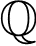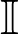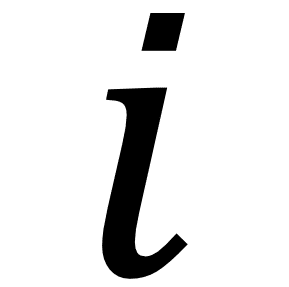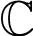# Numbers

## Living by Numbers

The Fourier transform is one of the most used and widespread applications of mathematics in electronic engineering, decomposing a signal into its constituent sine waves. It has applications in an enormous number of areas in everything from understanding electrical oscillations in alternating current to the modulation of electromagnetic waves. Engineering just wouldn’t be the same without it.

But, don’t worry, we’re not going to discuss the Fourier transform just yet. We’re going to discuss something without which the Fourier transform would be of no use whatsoever – numbers. And, something that puzzles people about numbers is their very nature – what kinds of numbers are there? How are they derived? And what we’re leading to is the what I like to think of as the ultimate type of number – the complex number.

Although you can perform a Fourier transform without complex numbers (well, you will see why I’m talking about this later) you could only retrieve magnitude or power information. To utilise the results fully, using complex numbers, you are able to retrieve phase information as well. Phase is just as important as magnitude, especially in filtering and image processing applications.

Fourier transforms are one example of many, another one is quantum mechanics – within which complex numbers are not just a convenience, they are a necessity – in effect laying the very foundations of physics.

## So, it sounds complex (no pun intended), but let’s see how complex numbers came about and how they related to the other numbers that you think you know about today.

I’m going to show you how there are different types of numbers, how they’re interrelated, and more interestingly how they’re defined in terms of each other. In order to do this I’m going to introduce a mathematical concept that you’re probably already familiar with, the set, which is described as a ‘well-defined’ collection of distinct objects.

For example, let’s take some everyday numbers 2, 3, and 4. They’re distinct objects in their own right, but when considered together like this, {2,3,4}, they form a set.
In this case the set is of size 3 and contains 3 distinct objects.

Set theory in itself is fascinating and pretty immense – in fact nearly all of mathematics can be defined in terms of set theory – but let’s leave that for another day.

Now, considering sets, I’m going to introduce another mathematical concept called closure that a set can have as a property. Formally if a set has closure under an operation (we’ll come to that in a second) then performance of that operation on the set will always produce a member of the same set.

An operation in this case is a calculation of some sort, and as we’re dealing with numbers it will be some sort of arithmetic calculation.
For example, simple arithmetic operations are addition, subtraction, multiplication, and division.## The Natural Numbers

Let’s start with the simplest numbers, the ones we use everyday for simple counting, the ones we use when we buy things, order lunch, or pay for things on the internet. Some people call them the ‘counting numbers’ but mathematically we call them the natural
numbers. Some people include zero in the natural numbers, some don’t, but for completeness I think we should too as we experience zero in our everyday counting.

If we imagine a set containing all of the natural numbers it has a special symbol, the nicely elaborate and suitably mathematical symbol ℕ.

Mathematically, ℕ = {0,1,2, …}

*Sometimes ℕ is written as ℕ0 when zero is included and ℕ* when zero is not

Now, here’s the thing, let’s explore whether this set is closed for some simple operations.

If we add any two natural numbers together, do we always get another natural number?
Well, how about 2 + 3, that’s 5 which is also a natural number. If we consider things carefully we see that we always get a bigger (or the same if we’re using zero) number which is always positive and a whole number so, yes, we always get a natural number – therefore we say that ℕ is closed under (the operation of) addition.

## Multiplication

Let’s do multiplication next as it’s really just a case of repeated addition and so is the natural next step from addition. It’s also my favourite, but you know.
So, back to the script, if we multiply any two natural numbers together then what we’re really doing is lots of repeated additions and since we know that addition is closed for ℕ then we can also conclude that so is multiplication.

## Subtraction

Let’s do subtraction next as it’s pretty simple and really is kind of the opposite of addition whilst not being as complicated as division (which is the opposite of multiplication, right?)
So, taking any two natural numbers, say 5 and 3, 5 – 3 = 2. Great, a natural number. Let’s try another, how about 10 and 20? Well, 10 – 20 is, well -10. Oops.

When we subtract a natural number from a smaller natural number we get a negative number. That’s strange (I was going to say that’s odd, but that would confused things…). We can’t have -2 cupcakes or have -5c in change at the local shop.

We’ve produced a number that isn’t natural!## The Integers

Okay, so we’ve produced a negative number – something that not everyone in history actually acknowledged existed! In order to cater for negative numbers we need to create a new set of numbers that includes both ℕ and what is essentially a negative reflection of ℕ.

We call this set of numbers the Integers and they also have a special symbol, ℤ. (The Z actually comes from the German word Zahlen meaning, suitably, “numbers”).

Mathematically, ℤ = {…, -3, -2, -1,0,1,2,3,…}

(With zero in this case being defined as neither negative nor positive.)

We can also say that ℤ is what’s termed a ‘superset’ of ℕ meaning that ℤ contains all of ℕ. Essentially, everything that is in ℕ is also in ℤ, and we can represent the relation like this:This kind of representation is known as a Venn diagram and is used to represent relations between items in sets. (I love the history of things, so it’s worth mentioning that Venn diagrams are named for John Venn who came up with the idea around 1880. They usually use circles but sometimes you’ll see generally curved lines.)

Let’s do some closure tests on ℤ.

Addition: we know that ℕ is closed so let’s trying adding some negative numbers – they can add up to either a negative or positive number which is inside of ℤ, so addition is closed.

Multiplication: we know that ℕ is closed so let’s trying multiplying some negative numbers or combinations of negative and positive numbers (integers) – there’s a rule for this kind of multiplication, remember your school days?

Positive * Positive = Positive
Positive * Negative = Negative
Negative * Positive = Negative
Negative * Negative = Positive

Nothing unusual there, we always get a whole number and it’s always positive or negative (or zero). So we can conclude that ℤ is closed under multiplication.

Subtraction: Well, this is how we got here in the first place and we’ve now covered the issue that subtracting two numbers from ℕ can become negative by extending our set to include the negative numbers. We can say that ℤ is closed under subtraction.

 Operation + * – Closed Yes Yes Yes

Division: Now, we come for the first time to division. Take any two numbers from ℤ and divide them by each other and what do you get? Well, how about 10 and 5? 10/5 is 2 so that’s ok. How about -2 and -2, well -2/-2 = 1 so we’re good there. But what happens with 5 and 10, 5/10 = ½ which isn’t in ℤ.Looks like we need to extend our set again.## The Rational Numbers

Okay, so we have a puzzle – we took an integer and divided it by another integer and got something that (sometimes) wasn’t an integer so we had to extend our set of numbers.

We have to extend our set to include numbers that are the result of two integers from ℤ being divided by each other.Considering the division operation carefully the result could be another integer or something that we now call a rational number (a number that has something other than zero after its decimal place) that belongs to the set ℚ.

For example, 10/5 = 2 which is an integer but as it’s a subset of the rational numbers is also a rational.
And with something like 5/2 = 2.5 we have something that isn’t an integer but is a rational. See what I mean?

So now we have a set of number, the rational numbers or ℚ that is closed under additional, multiplication, subtraction, and division. Great, so we’re done, right? Well, no. There are in fact more operations we can do to numbers as so far we’ve only considered the simple arithmetic ones. How about powers and roots?

Let’s try squaring some rational numbers, how about (4/9)2 for instance?

(4/9)2 = 4/9 * 4/9 = 16/81

Looks good, it’s still a rational number – i.e. one integer divided by another. Let’s try something a bit more esoteric, how about 2½ (or √2 as it can also be written).

It so happens that √2 cannot be represented as a rational number and written as one integer divided by another integer. There are many proofs for this (my favourite is the proof by infinite decent which uses a proof by contradiction). Check it out on Wikipedia.

Now, oops, √2 isn’t rational.
In fact, it’s a decimal that goes on forever, starting 1.41421356…

So now we have to make yet another set, as the numbers in the new set are not rational they will be called… wait for it… irrational numbers!## The Irrational Numbers (or ℝ \ ℚ)

Now we have another set of numbers, but there’s something very special about this set as they can’t be written as a complete ratio of two integers, i.e. a/b where a and b are both integers, something which makes them unique.

Due to this property they don’t include the rational numbers as a subset. In our diagram they have to go to the side and don’t overlap with the rational numbers.

Irrational numbers themselves cannot be written down fully and actually go on forever after their decimal place and their digits do not repeat. (This too can be proven, but we don’t have space here today.)

Inside the irrational numbers are also another set of special numbers that are called transcendental numbers.

Inside the irrational numbers are also another set of special numbers that are called transcendental numbers. Examples are numbers such as π and ε and various other irrational powers of reals. Proving that a number is transcendental is actually quite hard, but all transcendental numbers are themselves irrational therefore we must mention them here.We don’t have the space to go into the complete definition, but suffice it to say that transcendental numbers are not algebraic numbers (the set of algebraic numbers are denoted by the letter A).

What this means is that they are not roots of a (non-zero) polynomial equation with rational coefficients.

For example, an algebraic number is defined to exist as the solution to a non-zero polynomial equation such as,

0 = a0 + a1x + a2x2 + … + anxn

whereas a transcendental number cannot be expressed in this way. A little like the way that an irrational number cannot be expressed as a/b where a and b are integers.

You may think that this makes transcendental numbers a minority but in fact almost all complex (see later) and real numbers are transcendental numbers! Mind blown.

The irrationals are sometimes denoted by P or I but we’ll use the common nomenclature using the set complement operator “\” where A \ B is defined as the set of all elements that are members of A but not members of B. So they are in fact defined as A \ ℝ.

You’re probably wondering where the ℝ came from, right? Well, apologies, we had to leap ahead just one section to define what the irrationals and transcendental numbers were in terms of sets. You have probably guessed, but will find out in the next section.

Wow, this section took a little longer than I thought, I think we’ll have to go into transcendental numbers again in a future post. So, as we now have two distinct sets of numbers again, let’s group them all together…## The Real Numbers

Now we’re getting somewhere. We are able to define a set of numbers that includes all other sets of numbers – each having a distinct definition in terms of closures (or in the terms of the transcendental numbers algebraic operations).So, we’re there. Well, not quite. We still have further operations to consider – we did powers but what about roots? Are the reals closed when it comes to performing roots?

Let’s try a few examples. Firstly, √4, well that’s ok it’s just 2 or -2 which are both real. How about √2? Well, that’s irrational but also a real. Ok, how about √-1? Oops. We broke the real numbers – what exactly is the square root of -1? We can’t express it as a real number.

We need another set and we’re finally approaching the last pieces of the puzzle.## The Imaginary Numbers

A whole branch of mathematics is devoted to the study of imaginary numbers, stemming from the original solution to the question of just what is the square root of -1, or √-1.

Just to say that √-1 is defined as i where i 2 = -1.
So, if you wanted to calculate √-25 your answer would be 5i .

Imaginary numbers crop up in all kinds of places, especially in science and engineering, and are used both to simplify complex problems and of themselves have opened up many branches of mathematics in their own right.

Their very existence makes equations that were previously unsolvable now solvable in such a way that the non-existence of negative numbers made equations such as x + 5 = 0 previously impossible to solve.

They’re distinct in their own right and don’t have any subsets of our existing numbers so they will exist separate and distinct from the reals.

Once again we need to group things together, just like we did with the real numbers, and come to the last piece of the puzzle, the all defining class of numbers.

(For completeness, the number zero is consider both real and imaginary. Think on that.)## The Complex Numbers

Complex numbers contain the sets of all other sets of numbers. Our final diagram, though remember that the sizes of the sets are another matter altogether – we’re just showing how they relate to each other in terms of (set) membership.Consider some interesting facts that come from our diagram.

• Every number in mathematics is a complex number
• An integer cannot be transcendental
• An imaginary number isn’t a real number (kind of makes sense…)

And so on…

All complex numbers are written as,
a + bi
where a and b are real numbers and i is an imaginary number.

In your everyday life when you pay 1 euro for something, although you’re using an integer (or a rational if it’s say 1.05 euro, or 1 euro and 5 cent) you’re really using a complex number – with b equal to zero – thus removing the imaginary component of the complex number by making it zero!

Now, that’s cool and although it took a thousand years or so to get to this stage with the help of some very clever mathematicians I guess it was always meant to be.

Living by numbers, now…

## Extra Credit

Oh, wait, maybe you’re thinking there’s something even more encompassing, perhaps you’re thinking maybe we need something to explain something like the square root of an imaginary number like √-i ?

Well, that’s actually √2/2 – (√2/2) i.
I’ll leave the working out to you.

Complex numbers are closed for simple arithmetic operations as well as powers and roots and for algebraic expressions. The proofs are fun. Probably a definition of fun that you’ve not come across before, but fun nonetheless.

You can also think of complex numbers as two dimensional numbers that extend a traditional one dimensional number line out into two dimensional space. This is a fascinating way to think about complex numbers and will most likely the the subject of a future post too.

## Keywords:

Fourier, transform, complex, imaginary, numbers, rational, irrational, engineering, mathematics, transcendental